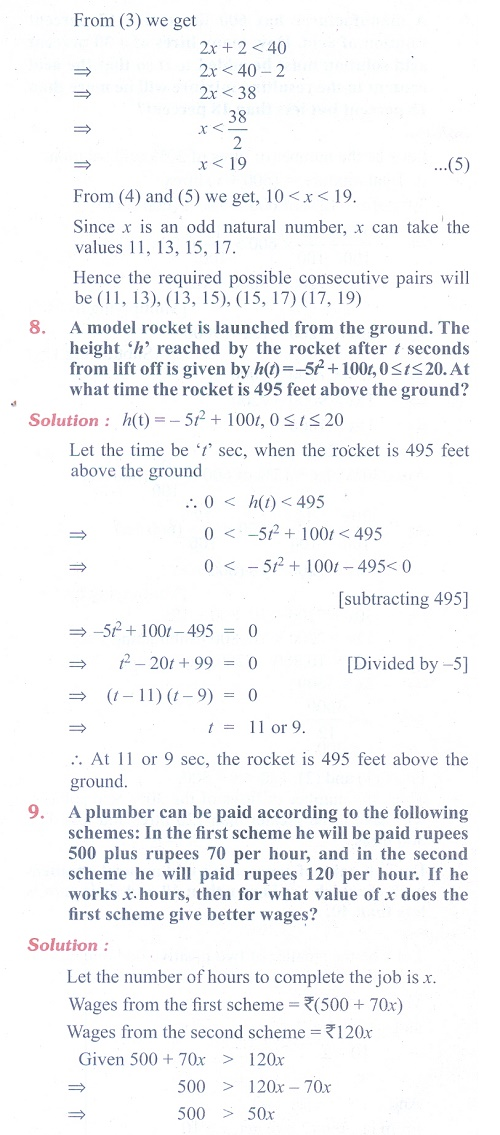Home | | Maths 11th std | Exercise 2.3: Linear Inequalities

# Exercise 2.3: Linear Inequalities

Maths Book back answers and solution for Exercise questions - Mathematics : Basic Algebra: Linear InequalitiesTags : Problem Questions with Answer, Solution | Basic Algebra | Mathematics , 11th Mathematics : Basic Algebra
Study Material, Lecturing Notes, Assignment, Reference, Wiki description explanation, brief detail
11th Mathematics : Basic Algebra : Exercise 2.3: Linear Inequalities | Problem Questions with Answer, Solution | Basic Algebra | Mathematics

Related Topics